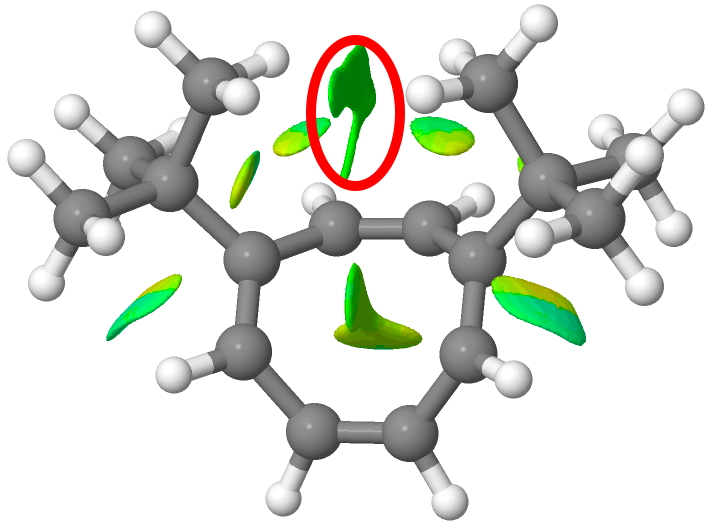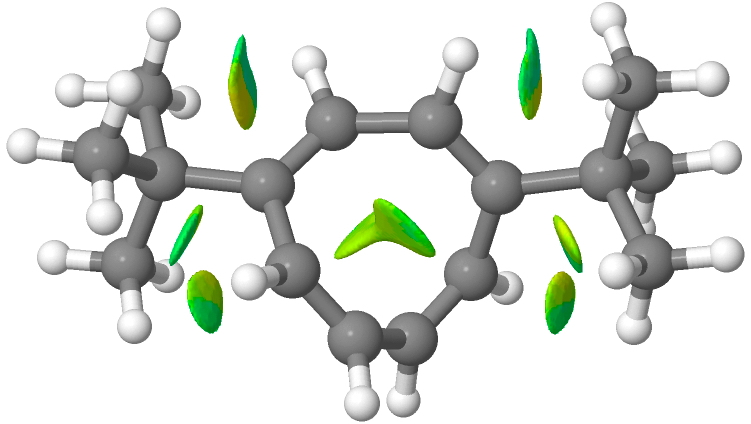## A molecular balance for dispersion energy?

The geometry of cyclo-octatetraenes differs fundamentally from the lower homologue benzene in exhibiting slow (nuclear) valence bond isomerism rather than rapid (electronic) bond-equalising resonance. In 1992 Anderson and Kirsch exploited this property to describe a simple molecular balance for estimating how two alkyl substituents on the ring might interact via the (currently very topical) mechanism of dispersion (induced-dipole-induced-dipole) attractions. These electron correlation effects are exceptionally difficult to model using formal quantum mechanics and are nowadays normally replaced by more empirical functions such as Grimme's D3BJ correction. Here I explore aspects of how the small molecule below might be used to investigate the accuracy of such estimates of dispersion energies.

The concentration of the two forms shown above can be readily estimated by NMR spectroscopy (the barrier is slow enough to allow peaks for both isomers to be integrated). This shows that the 1,6 form is present in greater concentrations than the 1,4 form, equivalent to a difference in free energy ΔΔG298 of 0.39 kcal/mol in favour of the former. Why is this? Because, it is claimed,  in the 1,6 isomer the two t-butyl groups are close enough to experience mutual dispersion attractions not experienced by the 1,4 form. This can be illustrated using the NCI display below for the two forms.Click for 3D. 1,6-isomer: Additional NCI interactions ringed in red.Click for 3D, 1,4 isomer.

Method Equilibrium constant, 298K ΔΔE ΔΔH298 ΔΔS298 ΔΔG298 Source
Experiment 1.93 1.14 -2.5 0.387 
B3LYP/Def2-TZVPP/CDCl3 (no dispersion) 1.906 0.05 0.00 +1.3 0.382 ,
B3LYP/Def2-TZVPP/CDCl3 (gd3bj dispersion) 8.36 0.75 0.66 +2.0 1.25 ,

This contains a contribution of RTLn 2 (= 0.410 kcal/mol = 1.04 in ΔS), where 2 is the symmetry number for a species with C2 rotational symmetry, to the 1,4-isomer only.

The interpretation of these results, as is often found, is non-trivial.

1. The relative concentrations of species in equilibrium equates with their relative free energies, ΔG298 and not ΔE (the difference in total energy computed using either quantum or molecular mechanics).
2. ΔG298  has a component derived from the entropy of the system, and this in turn has contributions from symmetry (numbers).  Only the 1,6-isomer has two-fold rotational symmetry for the lowest energy pose of the two t-butyl groups, and this contributes 0.41 kcal/mol to ΔG298. This aspect is not discussed in the original article.
3. The B3LYP/Def2-TZVPP DFT method predicts ΔΔE to be +0.05 kcal/mol without the inclusion of the D3BJ dispersion correction but +0.75 kcal/mol with. One might approximately equate the latter to the contributions ringed in red in the NCI distributions shown above. The enthalpies (where ΔΔE is corrected for zero point energies) are very similar.
4. Conversion to ΔG298 involves use of the vibrational frequencies to obtain the entropy; here one encounters a difference between the two double bond isomers. The lowest energy vibration for C2-symmetric 1,4 is 23 cm-1, whereas that for the 1,6 is only 7 cm-1 (a value which also depends on round-off errors and accuracies in the calculation). These errors in the RRHO (rigid-rotor-harmonic-oscillator) approximations makes meaningful calculation of ΔS298 and hence ΔG298 problematic at this small energy difference level. In both cases, this approach suggests that the entropy of the 1,6 form is slightly larger than the 1,4 isomer, whereas the reverse is apparently true by experimental measurement. It might all boil down to those low-frequency vibrations!

So we may conclude that whereas the dispersion uncorrected method gets the right answer for the equilibrium constant for probably the wrong reasons, inclusion of a dispersion correction would get the right answer were it not for the error in the entropy. Agreement with experiment would be obtained if the calculated entropy difference were to be -0.9 kcal/mol K-1 instead of +2.0. Thus the 1,6 isomer has the two t-butyl groups weakly interacting (red circle above), which intuition tends to suggest would reduce the entropy (reduce the disorder) of the system and not increase it.

At least in this relatively small molecule, we now have a handle for estimating these sorts of effects in terms of variables such as the basis set used, the energy Hamiltonian (e.g. type of functional etc) and of course the dispersion correction.

## References

1. J.E. Anderson, and P.A. Kirsch, "Structural equilibria determined by attractive steric interactions. 1,6-Dialkylcyclooctatetraenes and their bond-shift and ring inversion investigated by dynamic NMR spectroscopy and molecular mechanics calculations", Journal of the Chemical Society, Perkin Transactions 2, pp. 1951, 1992. http://dx.doi.org/10.1039/P29920001951
2. S. Grimme, S. Ehrlich, and L. Goerigk, "Effect of the damping function in dispersion corrected density functional theory", Journal of Computational Chemistry, vol. 32, pp. 1456-1465, 2011. http://dx.doi.org/10.1002/jcc.21759
3. Henry S Rzepa., "C 16 H 24", 2016. http://dx.doi.org/10.14469/ch/191875
4. Henry S Rzepa., "C 16 H 24", 2016. http://dx.doi.org/10.14469/ch/191876
5. Henry S Rzepa., "C 16 H 24", 2016. http://dx.doi.org/10.14469/ch/191874
6. Henry S Rzepa., and Henry S Rzepa., "C 16 H 24", 2016. http://dx.doi.org/10.14469/ch/191880